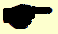# Mock exam paper B P2 Ordinary Level

 Name Class Teacher SchoolPRE – JUNIOR CERTIFICATE EXAMINATION, 2012

MATHEMATICS – ORDINARY LEVEL – Paper 2 (300 marks)

Time: 2 hours

Attempt ALL questions. Each question carries 50 marks.

Answers and supporting work should be written in the boxes provided.

Extra paper and graph paper can be obtained from the Superintendent, if needed.

The symbol indicates that supporting work must be shown to obtain full marks.

____________________________________________________________

Make and model of calculator used

 Question Mark Adv. Exam. 1 2 3 4 5 6 Total Grade

For Superintendent / Examiner use only:

(b) A car leaves Cork at 08:45 and arrives in Limerick at 10:00.

(i) How many hours and minutes did the journey take?

(ii) The car travelled at an average speed of 80 km/hr. Calculate the distance the car travelled?

(iii) The return journey takes 24 minutes longer than the earlier journey. If the car returns back in Cork at 22:30 at what time did it leave Limerick?

(c) A sitting room has a floor plan as shown.

8m

4m

2m

1m

1. Find, in, the area of the sitting room.

(ii) The owner wants to carpet the floor. The carpet she picks costs €32 per square metre. Calculate the cost of the carpet required.

(iii) The carpet shop charges €11 per square meter to lay the carpet. Calculate the total cost putting down new carpet in the room.

2. (a) A ball in the shape of a sphere has radius 21cm. Taking, find in, the volume of the ball.

(b) A cube has side length 5cm.

.

(i) Find the volume of the cube, in .

(ii) A rectangular box is filled with 48 of these cubes. Find the volume of this rectangular block, in.

(iii) This rectangular block is 25cm long, 15cm wide and 16cm, find the surface area of the block, in.

(c) A factory imports metal in the shape of cylindrical rods. Each rod has a radius of 3cm and length 40cm.

(i) Find, in terms of , the volume of each metal rod.

(ii) The cylindrical rod is melted down and made into spheres.

If each sp...